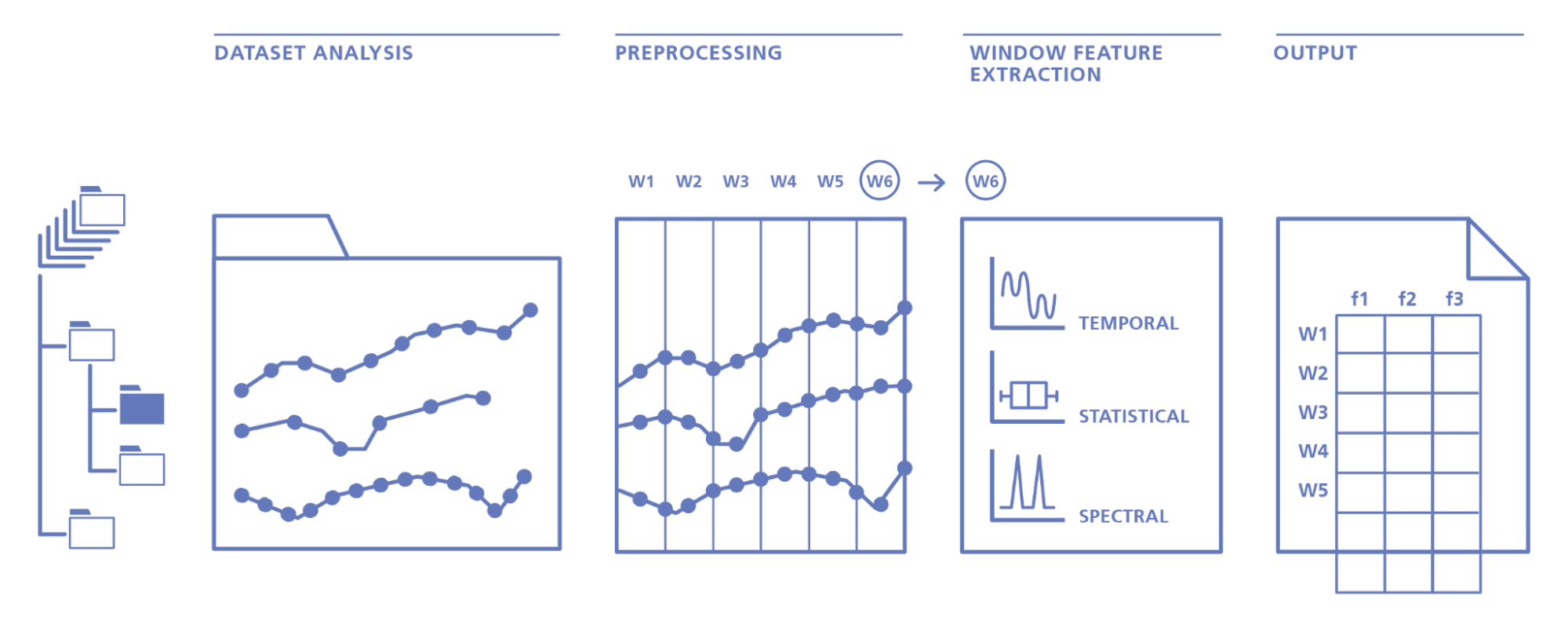Course Content

# Data Preprocessing

2. Processing Quantitative Data

3. Processing Categorical Data

4. Time Series Data Processing

Data Preprocessing

##Feature Extraction from Time Series

The last data type for which we will look at the feature extraction process is time series data.

What data can we extract from such datasets? Feature extraction from time series refers to the process of identifying and extracting important patterns, trends, and features from a time series dataset. This is important for various applications such as forecasting, anomaly detection, and classification. Feature extraction techniques can help reduce the dimensionality of time series data and extract meaningful information that can be used in machine learning models for predictive analysis.There are several methods for feature extraction from time series data. Here are four common methods:

1. Statistical features: this involves computing various statistical properties of the time series, such as mean, variance, skewness, kurtosis, and so on. These features are useful for detecting trends and patterns in the data.
2. Fourier transforms involve transforming the time series into the frequency domain using a Fourier transform. This allows us to identify periodic patterns and cyclical trends in the data.
3. Wavelet transforms involves transforming the time series into the time-frequency domain using a wavelet transform. This allows for the identification of localized features and trends in the data.
4. Autoregression: this involves modeling the time series as a linear combination of its past values. This allows for the identification of patterns and trends in the data over time.

Let's move on to an example. In plasmonics research, Fourier transforms analysis is used to study the behavior of plasmons, which are electromagnetic waves that propagate on metal surfaces. Plasmons have applications in various fields, including biomedical imaging, sensing, and optical communication.

In plasmon behavior analysis, Fourier transforms can be used to extract the frequencies of interest from a time series of plasmon resonance data.

We can use the `numpy` library to perform a Fourier transform on plasmon resonance data. The code will read in a `csv` file of plasmon resonance data and perform a Fourier transform to extract the amplitudes and phases of the frequencies of interest: